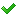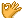•闪存
•博客
• 发言小组
• 投递新闻
• 提问博问
• 添加收藏
•文库

2

##10C语言中关于函数的一些应用。

include<stdio.h> include<math.h> double f(double x); int main(void) { int n,i; double result, x; sca
8

1

##我编了一套代码，但是根本输出不了东西，这是咋回事？

include <stdio.h> int main(void) { int n,i,count,a; count=0; while(scanf("%d",&n)!=EOF) for(i=1;i<=n
7

1

##输入多组数据，每组数据包含整数啊a，b，包含0 ，0则停止测试

include<stdio.h> int main(void) { int a,b; while(scanf("%d %d",&a,&b)!=EOF) {if(a==0&&b==0) break; e